# Top 20 SAT Math Formulas | You Need to Learn These to Do Well in the Test

The SAT has two math sections - one that doesn't require a calculator and is purely based on your math knowledge, and the other that allows you to use a calculator - see best calculators here - since you're going to solve problems with SAT math formulas.

Many students find SAT's math section the hardest because they come unprepared (see the best SAT prep books reviews). However, if you brushed up on the math topics and formulas needed in answering the solutions presented on the exam, you'd realize that all of the questions are subjects you learned in high school.

Below are all the SAT math formulas you need to know and the main mathematics topics included in the SAT:

## What Kind of Math is Used in SAT?

The first math section has 20 questions, which you must complete in 25 minutes without calculators. The second and final math section has 38 questions, which you must complete in 55 minutes with calculators.

Math is categorized into four categories called "domains":

1. Heart of Algebra

• 33% of the test fall under the Heart of Algebra domain

• This domain tests your ability to analyze and solve linear equations and systems of linear equations.

• Questions covered here involve absolute value, a system of equations, linear equations, linear graphing equations, and systems of inequalities.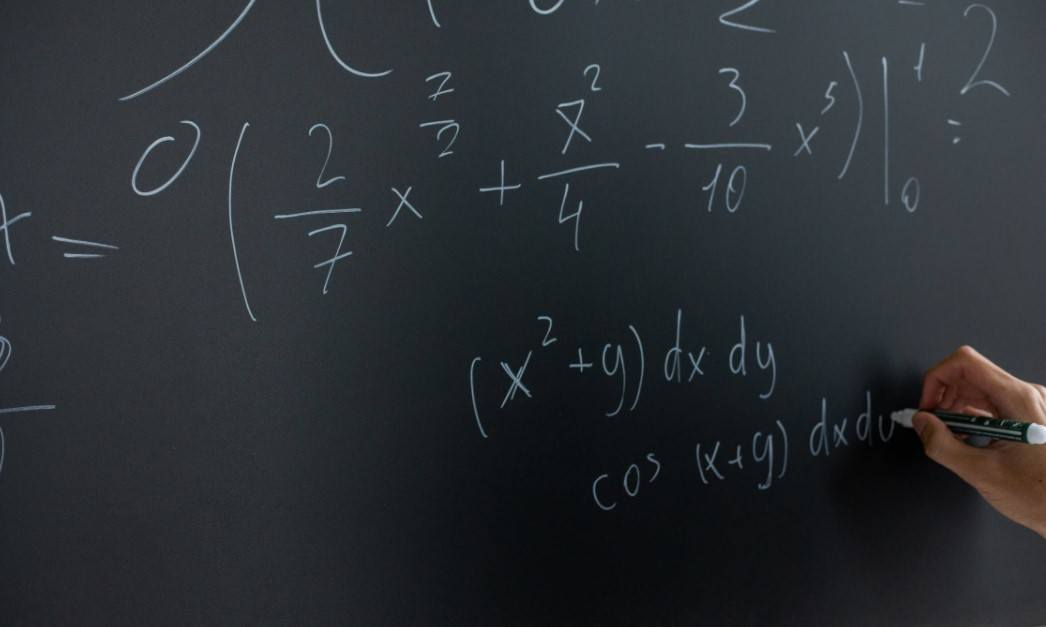2. Problem Solving and Data Analysis

• This domain tests your ability to use your math understanding to solve real-world problems.

• You might be asked to create an equation from a word problem, use percentage in various contexts, or use rates, ratios and proportions.

• Students may be asked to convert units (for example: converting kilometer per hour to meters-per-second), interpret the slope of the line, compute probability and interpret probability, and so on.• This domain tests your skills in "advanced math" and ensures you are ready to take pre-calculus and calculus classes in college.

• You'll face problems that focus on solving quadratic equations, understanding graphs of quadratic functions, and using quadratic functions.

• Other topics in this domain include polynomial factors, linear and quadratic systems, radicals and rational exponents, nonlinear equations, and isolating quantities.• 10% of the test falls in this category.

• This domain tests your knowledge of essential geometric and trigonometric concepts and the Pythagorean theorem.

• Example of questions involves area, volume, right triangle trigonometry, complex number system, and circles.

• You might also face problems relating to the concepts of angles, triangles, and lines, including right triangle geometry and word problems, complex numbers, circle theorems, and circle equations.## What Formulas are Given During the SAT Math Section?

If you're wondering if the SAT provides math formulas, you'll be glad to know that you have a reference sheet that looks like this: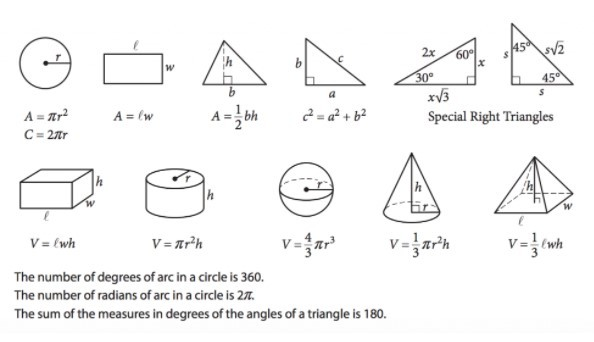You'll find this sheet at the beginning of both math sections (the calculator and no calculator section), so you really don't need to memorize them anymore. Just make sure you understand the concepts to be able to answer these questions.

As you can see from the reference sheet, there are 12 geometric formulas for triangles, circles, areas, and volume.

• Area of a circle (A= πr²)

• Circumference of a circle (C=πd or 2πr)

• Area of a rectangle (A=lw)

• Area of a triangle (A= 1/2 bh)

• The Pythagorean Theorem (a²+b²=c²)

• Isosceles right triangles (H = s√2)

• Special Right Triangle in 30/60/90 degrees

• The volume of a rectangular solid (V=lwh)

• The volume of a cylinder (V=πr²h)

• Sphere volume (V=4/3 πr³)

• Cone volume (V=1/3πr²h)

• Pyramid volume (V=1/3lwh)

With the reference sheet above, you should be able to calculate the respective algebra and trigonometry formulas more easily. Just familiarize yourself with them and practice each formula.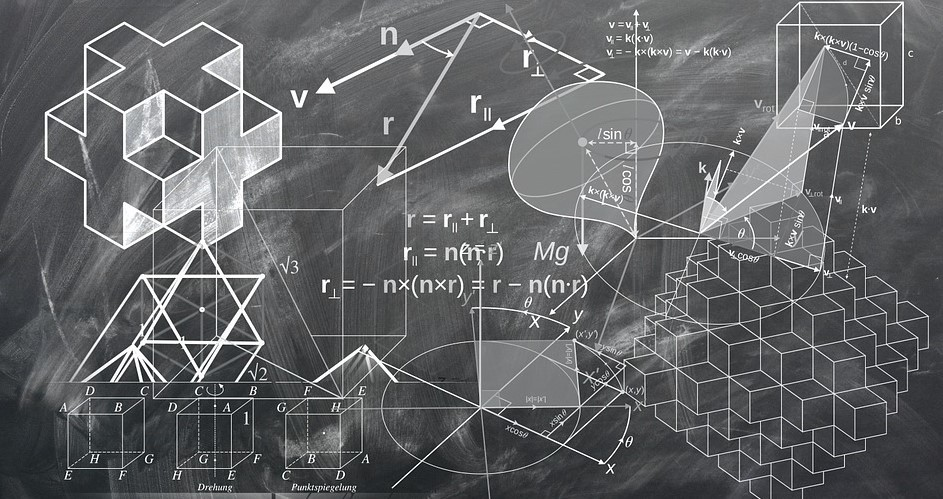## Top 20 SAT Math Formulas You Must Know

The geometric formulas above would only make up 10% of the math test questions, so even if you've answered all of them perfectly, the other 90% will still make a bigger chunk of your score. Here is where I recommend you focus most of your test prep and practice these 20 formulas:

Memorize the word SOHCAHTOA, which refers to "Sine equals Opposite over Hypotenuse," "Cosine equals Adjacent over Hypotenuse, and "Tangent equals Opposite over Adjacent."

### 1. Distance Formula

Learn how to find the distance from A to B. Given two points, A(x1,y1), B(x2,y2), find the distance between them. You can use the Pythagorean Theorem to solve this kind of problem.

### 2. Lines and Slope Formula

When it comes to lines and slopes, the formula you need to know doesn't just find the length of a line. Instead, you have to memorize the formula for the following:

• Slope-intercept form or how to present the equation of a line (y = mx + b)
• Point-slope form (y - y1 = m (x-x1)
• Slope of a line: (y1-y2)/(x2-x1)

Note that parallel lines have the same slope, while perpendicular lines have slopes that are negative reciprocals.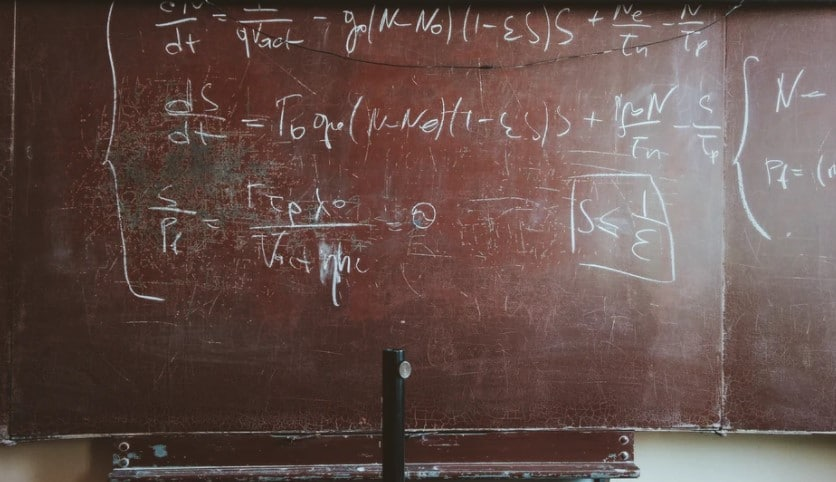### 3. Averages

• Average = sum of terms/number of terms
• Average speed = total distance/total time
• Sum = average x (number of terms)

The standard form is ax2 + bx +c = y, where you'll set y equal to 0, and solve for x by factoring or using the formula below:

• (x2-y2)=(x+y)(x-y)
• x2+2xy+y2=(x+y)2
• x2-2xy+y2=(x-y)2

### 5. Probability

• Probability = number of desired outcomes/number of total outcomes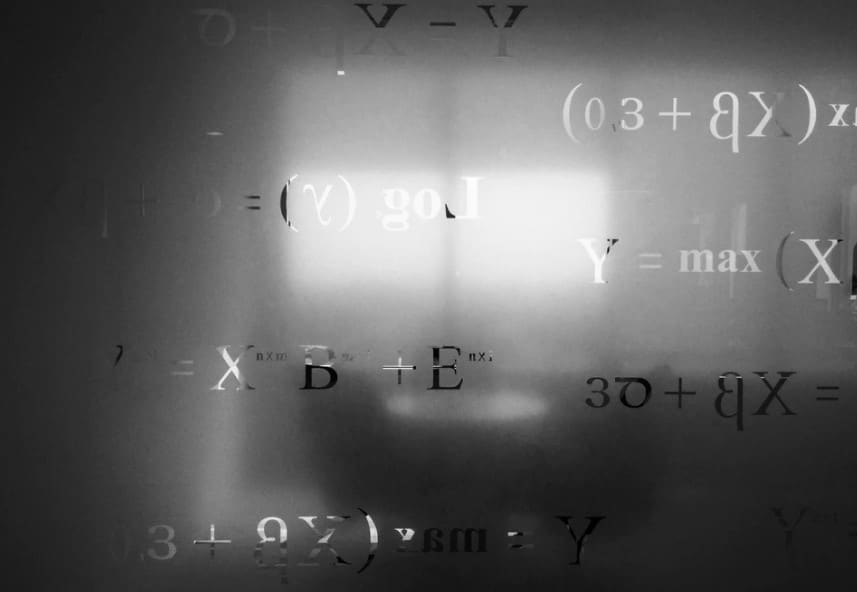### 6. Exponents

• Multiplication: a n x a m = a n+ m

### 7. Triangles

• all triangles: a2 + b2 = c2
• Isosceles right triangle: x, x, x√2
• 30-60-90 Triangle: x,x√3,2x

Third side rule: (a-b) < c < (a+b) if c represents the third side, then b and a represent the lengths of the other two sides.

• Area of a triangle: A = 1/2bh
• exterior angle of a triangle: d = a + b
• Area of a trapezoid: A = 1/2h (a+b)

### 8. Circles

• Equation of a circle: ( x - h )^2 + ( y - k )^2 = r^2
• Length of arc in a circle: θ times the radius of a circle
• Area of a Sector of Circle = (θ360º)×πr2
• A = πr2
• Circumference of a circle: C = πd = 2π### 9. Cube and Squares

• Surface Area of a Cube = 6s2
• Difference of squares: a2 − b2 = (a + b)(a − b)

### 10. Rectangular and Cylinders

• volume of a rectangular solid: V = lwh
• surface area of a rectangular solid: SA = 2lw + 2wh + 2lh
• Diagonal of a rectangular solid: d2 = l2 + w2 + h2
• Volume of a right cylinder: V = πr2h
• surface area of a right cylinder: SA = 2πr2 + 2πrh

### 11. Cross Multiplication

• If a/b = c/d, then ad = bc.

### 12. Absolute Value

• If x = 4,then x = 4 or x = -4
• If x > 4,then −4<x<4
• If X > 4,then x<−4 or x>4### 13. Linear and Quadratic Functions

• linear: y=mx + b
• quadratic: y= ax2 + bx+ c

### 14. Percentage

• Percent/100 = part/whole (can also be turned into an algebraic equation like 40% of what is 20?
• Percent change = amount of change/original

### 15. Angles

• The sum of any number of angles that form a straight line is 180°.
• The sum of any number of angles around a point is 360°.
• vertical angles: a = d and b = c
• transversals:
• a = d = e = h
• b = c = f = g
• c + e = 180° and d + f = 180°

### 16. Trigonometric Relationships

• How to find the sine of an angle: sin(x)= Measure of the opposite side to the angle/measure of the hypotenuse
• How to find the cosine of an angle: cos(x)= Measure of the adjacent side to the angle/measure of the hypotenuse
• How to find the tangent of an angle: tan(x)= Measure of the opposite side to the angle/measure of the adjacent side to the angle### 19. Interest

• Simple interest: A=Prt
• Compound interest: A=P(1+r/n) nt

### 20. Imaginary numbers and complex numbers

An imaginary number is a complex number defined as a real number multiplied by the imaginary number I, where "i" is defined as the square root of a negative one.## Don't Be Scared of SAT Math Formulas

The SAT Math Test isn't really that complicated. If you come prepared on test day (check out the best SAT prep courses), you'll understand that the two sections both measure your ability to apply mathematical concepts, which you'll need in solving problems you might see in college and in your career.

The formulas won't work if you don't understand the rules of absolute value, the rules of inequalities, and the formula for slope-intercept form.

Armed with a calculator and the right set for Math formulas and knowledge, you'll be ready to answer all the math questions on your SAT test day. After all, most of the concepts included here are trig, geometry, algebra, probability topics you've been learning from middle school to high school. You got this.

``` { "@context": "https://schema.org", "@type": "FAQPage", "mainEntity": [{ "@type": "Question", "name": "What Kind of Math is Used in SAT?", "acceptedAnswer": { "@type": "Answer", "text": "The first math section has 20 questions, which you must complete in 25 minutes without calculators. The second and final math section has 38 questions, which you must complete in 55 minutes with calculators.Math is categorized into four categories called \"domains\": 1. Heart of Algebra 33% of the test fall under the Heart of Algebra domain This domain tests your ability to analyze and solve linear equations and systems of linear equations. Questions covered here involve absolute value, a system of equations, linear equations, linear graphing equations, and systems of inequalities.2. Problem Solving and Data Analysis This domain tests your ability to use your math understanding to solve real-world problems. You might be asked to create an equation from a word problem, use percentage in various contexts, or use rates, ratios and proportions. Students may be asked to convert units (for example: converting kilometer per hour to meters-per-second), interpret the slope of the line, compute probability and interpret probability, and so on.3. Passport to Advanced Math This domain tests your skills in \"advanced math\" and ensures you are ready to take pre-calculus and calculus classes in college. You'll face problems that focus on solving quadratic equations, understanding graphs of quadratic functions, and using quadratic functions. Other topics in this domain include polynomial factors, linear and quadratic systems, radicals and rational exponents, nonlinear equations, and isolating quantities.4. Additional Topics in Math 10% of the test falls in this category. This domain tests your knowledge of essential geometric and trigonometric concepts and the Pythagorean theorem. Example of questions involves area, volume, right triangle trigonometry, complex number system, and circles. You might also face problems relating to the concepts of angles, triangles, and lines, including right triangle geometry and word problems, complex numbers, circle theorems, and circle equations." } },{ "@type": "Question", "name": "What Formulas are Given During the SAT Math Section?", "acceptedAnswer": { "@type": "Answer", "text": "You'll find this sheet at the beginning of both math sections (the calculator and no calculator section), so you really don't need to memorize them anymore. Just make sure you understand the concepts to be able to answer these questions. As you can see from the reference sheet, there are 12 geometric formulas for triangles, circles, areas, and volume. Area of a circle (A= &#x3C0;r&#xB2;) Circumference of a circle (C=&#x3C0;d or 2&#x3C0;r) Area of a rectangle (A=lw) Area of a triangle (A= 1/2 bh) The Pythagorean Theorem (a&#xB2;+b&#xB2;=c&#xB2;) Isosceles right triangles (H = s&#x221A;2) Special Right Triangle in 30/60/90 degrees The volume of a rectangular solid (V=lwh) The volume of a cylinder (V=&#x3C0;r&#xB2;h) Sphere volume (V=4/3 &#x3C0;r&#xB3;) Cone volume (V=1/3&#x3C0;r&#xB2;h) Pyramid volume (V=1/3lwh) With the reference sheet above, you should be able to calculate the respective algebra and trigonometry formulas more easily. Just familiarize yourself with them and practice each formula." } }] } ```
##### Leonard Haggin

I created this site to help students like you learn from the experiences my team had learned during our extensive academic careers. I am now studying Law at Stanford, but I also make time to write articles here in order to help all you fellow students advance in your academic careers and beyond. I hope our efforts on Study Prep Lounge will arm you with the knowledge you need to overcome whatever trial or test you find in front of you.